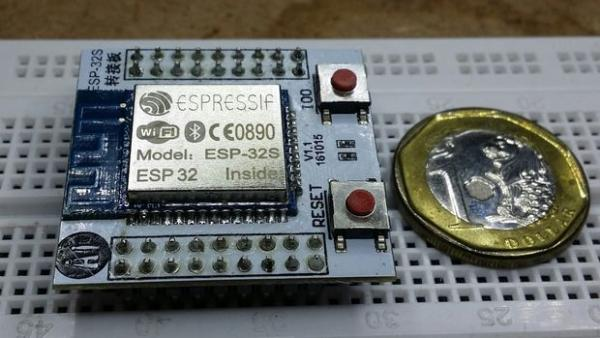# ESP32 Arduino: LED PWM fading

The objective of this post is to explain how to fade a LED with the ESP32, using the LED PWM functionalities.

Introduction

The objective of this post is to explain how to fade a LED with the ESP32, using the LED PWM functionalities of the microcontroller. I will be using DFRobot’s FireBeetle ESP32 board to perform the tests. Since the board as a built in LED, no external hardware will be needed.

Note that at the time of writing, the commonly used analogWrite Arduino function was not yet available for the ESP32 Arduino environment support . Thus, we will need to go to lower level functions in this tutorial. Nevertheless, we will also have more control and flexibility in the PWM functionality, which is good.

In terms of hardware, the LED PWM of the ESP32 is composed of 16 independent channels, with configurable duty cycles and wave periods . The accuracy of the duty cycle can be configured until 16 bits of resolution . The setup

In the first portion of the code, we will specify some global configuration constants. The first one will be the frequency of the PWM signal generated to control the LED. We will use a value of 5000 Hz. Note however that the maximum values for the frequency are not yet very clear and in the header file of the functionality we are going to use is stated that the maximum frequency depends on the resolution chosen.

We are algo going to specify the LED PWM channel and the resolution of the PWM duty cycle, in bits. Also from the header file, we can see that we can choose a channel from 0 to 15 and a resolution between 1 and 16 bits, which is coherent with the values mentioned on the introductory section. We will use channel 0 and a resolution of 8 bits.

Now, in the setup function, we will configure the LED PWM functionalities. First, we need to setup the channel, frequency and resolution we specified. To do so, we call the ledcSetup function, which receives as input the 3 previously mentioned parameters, in the same order.

 1 `ledcSetup(ledChannel, freq, resolution);`

Note however that the channel is not the pin where we will control the LED. So, we will need to attach channel 0 (which was the one we defined) to the digital pin where we want the PWM signal to be generated.

In our case, we want it to be generated on the pin that is connected to the on board LED. As we’ve seen in this previous tutorial, we can use the LED_BUILTIN constant to get the number of the pin connected to the LED on our FireBeetle ESP32 board.

To attach the pin to the PWM channel, we call the ledcAttachPin function, passing as arguments the number of the GPIO pin and the PWM channel previously defined.

 1 `ledcAttachPin(LED_BUILTIN, ledChannel);`

The full setup function is shown bellow, along with the constants definition.

 1 2 3 4 5 6 7 8 9 10 `int` `freq = 5000;` `int` `ledChannel = 0;` `int` `resolution = 8;` `void` `setup() {` `  ``ledcSetup(ledChannel, freq, resolution);` `  ``ledcAttachPin(LED_BUILTIN, ledChannel);` `}`

## Main loop

We will write the code to control the duty cycle values of the signal in our Arduino main loop. But the most important function will be the one that allows us to specify the duty cycle value. This is the ledcWrite function, which receives as first input the PWM channel (not the number of the GPIO) and as second input the value for the duty cycle.

READ  4x4x4 LED-cube based Arduino and Flower protoboard

Since we defined a 8 bits resolution, we can specify a duty cycle value between 0 and 255 (2^8 -1). So, we will iterate between these values in two loops, one ascending and other descending. You can check bellow the full source code, which already includes the call for the ledcWrite function and these two loops.

 1 2 3 4 5 6 7 8 9 10 11 12 13 14 15 16 17 18 19 20 21 22 23 24 `int` `freq = 5000;` `int` `ledChannel = 0;` `int` `resolution = 8;` `void` `setup() {` `  ``ledcSetup(ledChannel, freq, resolution);` `  ``ledcAttachPin(LED_BUILTIN, ledChannel);` `}` `void` `loop() {` `  ``for` `(``int` `dutyCycle = 0; dutyCycle <= 255; dutyCycle++){` `ledcWrite(ledChannel, dutyCycle);` `delay(7);` `}` `for` `(``int` `dutyCycle = 255; dutyCycle >= 0; dutyCycle--){` `     ``ledcWrite(ledChannel, dutyCycle);` `     ``delay(7);` `  ``}` `}`

## Testing the code

To test the code, just upload it with the Arduino IDE. Then, you should see the on board LED fading. Check the video bellow for the expected result.

## References

Read more: ESP32 Arduino: LED PWM fading

#### This Post / Project can also be found using search terms:

• arduino esp32 fading an led
• esp32 rgb android
• arduino mega PWM fade
• use arduino pwm to power led

© 2013 Powered By Wise Technologies, Use Arduino for Projects | Sitemap | Privacy Policy

###### Read previous post:##### ESP32 With Arduino IDE

Christmas 2015 yours truly was one of the lucky few in the world to receive the ESP32 for experimenting. The...

Close